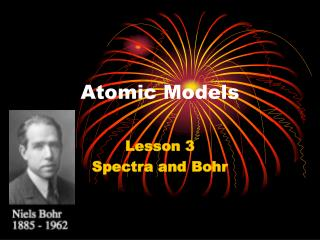# Atomic Models - PowerPoint PPT PresentationDownload PresentationAtomic Models

Atomic ModelsDownload Presentation## Atomic Models

- - - - - - - - - - - - - - - - - - - - - - - - - - - E N D - - - - - - - - - - - - - - - - - - - - - - - - - - -
##### Presentation Transcript

1. Atomic Models Lesson 3 Spectra and Bohr

2. Objectives • You will be able to – Explain the conditions necessary to produce a continuous spectrum and a line spectrum. – Explain that each element has a unique line spectrum. – Calculate the photon energies emitted and absorbed as electrons move in the hydrogen nucleus.

3. Review: The Story So Far: • The Billiard Ball Model all matter is made of atoms atoms of different elements are different atoms can not be split • The Raisin Bun Model charge to mass ratio experiment atoms are made of +ive and -ive matter the –ive matter is much smaller than the +ive the electron is inside the nucleus • J.J Thomson goldfoil Experiemnt atoms have a small, dense nucleus electrons orbit outside the nucleus in circles problems Dalton Thomson Rutherford

4. Review: Cont. • Recall that Rutherford's main problem was that if electrons are to travel in circles, classical physics says they have a centripetal acceleration. • And Maxwell said that if an electron accelerates, it gives off energy in the form of EMR. • This means that all matter would continuously give off EMR.

5. If the electron loses EMR energy, it loses velocity. • If it loses velocity, it's radius gets smaller. • And sooner or later, the atom would collapse!

6. Bohr Model • This problem was solved by Bohr. • He postulated that an atomic system can exist in any one of several states which involve no emission of radiation. • Any emission or absorption of EMR corresponds to a sudden transition between two such energy states. – Analogy is that of gravitational potential energy and a box on a shelf. Changing shelves requires energy but moving on the same shelf does not change the potential energy.

7. Quantized energy • Bohr stated that the EMR given off or absorbed would be in accordance with Planck’s energy quanta. E = hf • So the energy emitted (hf) was equal to the change in the energy levels Ef – Ei hf= Ef - Ei • Where: Ei is the energy of the initial state and Ef is the energy of the final state.

8. Bohr’s Model 1. Electrons orbit the nucleus only at certain distances from the nucleus. These distances are all multiples of the smallest distance an electron can orbit in. Therefore, electron orbits are quantized. 2. Each orbit corresponds to a certain energy level. Electrons can only exist in these particular energy levels. 3. An electron jumps from one level to another by emitting or absorbing energy equal to the distance between the energy levels.

9. Implications Bohr's model had some unique implications: • If the electrons stay in the same energy level, they will not emit energy. This flew in the face of everything classical physics had said. • More on this later…

10. Bohr's Model for Hydrogen • Let's look at this model for the simplest of all atoms: hydrogen. • The single electron can only occupy certain orbitals. These are called energy levels. • This atom has 3 energy levels. They are numbered 1-3.

11. Hydrogen: Con’t. • The e- only emits EMR when it moves down in energy levels. • To jump up a level EMR absorbs light.

12. Bohr Model, Con’t • Each orbital has its own number. The lowest orbital is called the ground state. This is the lowest possible energy of the electron. • Each orbital above that is an excited state. ground: n = 1 1st excited n = 2 2nd excited n = 3 etc... *note: although I draw it that way, the orbitals are not equally spaced

13. Changing Levels • The jumps produced spectral lines when emitting EMR and leave black spots on the spectrum when absorbing EMR. • The spectral lines for a element are like a finger print, unique to that element alone.

14. Spectroscopy • What can you conclude about the compostion of the Sun from the spectra given above? Explain your reasoning. See p. 781

15. Spectroscopy • A single jump b/t any two energy levels of hydrogen gives a red line (this is the lowest energy, therefore it emits light with lowest freq). • A jump of two levels gives green. • A jump of three levels gives blue. • A jump of four levels gives violet. • It was later discovered that two other invisible lines were present: these were UV light. These make up a 5th and 6th orbital.

16. Describing “Jumps” • The jumps in energy level are often shown in an energy level diagram.

17. Alternate Descriptions • In other cases, the ground state (n = 1) is the zero level for energy.

18. Strengths & Weaknesses • Strengths of the Bohr Model: 1) Explains the emission and absorption spectra of hydrogen 2) Explained the repetition of chemical properties on the periodic table • Weaknesses: 1) Electrons don't actually go in set paths...more on this at the end of the term!

19. Hydrogen Energies: Examples 1. Using the diagram for hydrogen, determine the frequency of the photon emitted as an electron moves from the fourth level to the third level. 2. Note: The energies are always stated as negative values. These are the energies that the electron must absorb so that it will leave the atom. (Ionization Energy). What is the ionization energy of the third level? 3. Determine the wavelength of the EMR absorbed as an electron moves from the first to the third energy level.